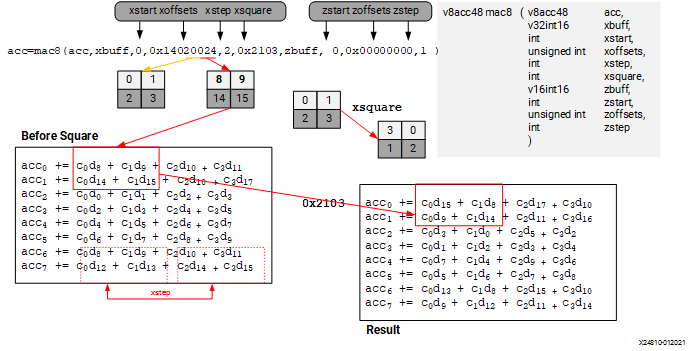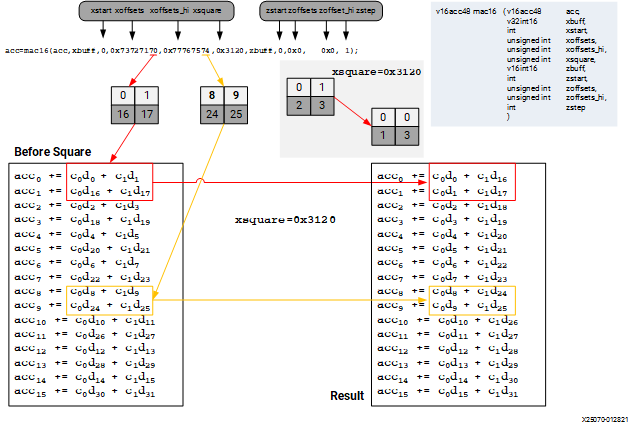# MAC on 16x16 bits - 2023.1 English

## AI Engine Kernel and Graph Programming Guide (UG1079)

Document ID
UG1079
Release Date
2023-06-23
Version
2023.1 English

An example of MAC with int16 `X` buffer and int16 `Z` buffer is as follows. Note that the permute granularity for `X` buffer is 32 bits. The `start` and `step` parameters are always in terms of data type granularity. To get to a 16-bit index, you need to multiply them by 2.

The `xoffsets` parameter comes as a pair. The first hex value is an absolute 32 bits offset and picks up 2 x 16 bits values (index, index+1) in the even row. The second hex value is offset from first hex value plus 1 (32 bits offset) and picks up 2 x 16 bits values in the odd row. So the hex value `0x24` in `xoffsets` selects index 8, 9 for even row and index 14, 15 for odd row from `xbuff`:

``````even: 2 * 4 -> get indices [8, 9]
odd: 2 * ( 2 + 4 + 1 ) -> get indices [14, 15]``````

Similarly, the hex value `0x00` in `xoffsets` selects index 0, 1 for even row and index 2, 3 for odd row from `xbuff`.

There is another `xsquare` parameter to perform 16 bits granularity twiddling after the main permute. It will give additional contribution to the index in a 2 by 2 matrix recurring across the 8x4 matrix compute given by MUL8 in int16 x int16 mode.

For example, `xsquare` value `0x2103` (see from lower hex value to higher hex value) puts index 3, 0 in the even row and index 1, 2 in the odd row. How the `xsquare` parameter works can be seen in the center of the following figure.

Figure 1. MAC8 on int16 x int16 TypeThe following figure is an example of `mac16` intrinsic of int16 and int16.

Figure 2. MAC16 on int16 x int16 Type